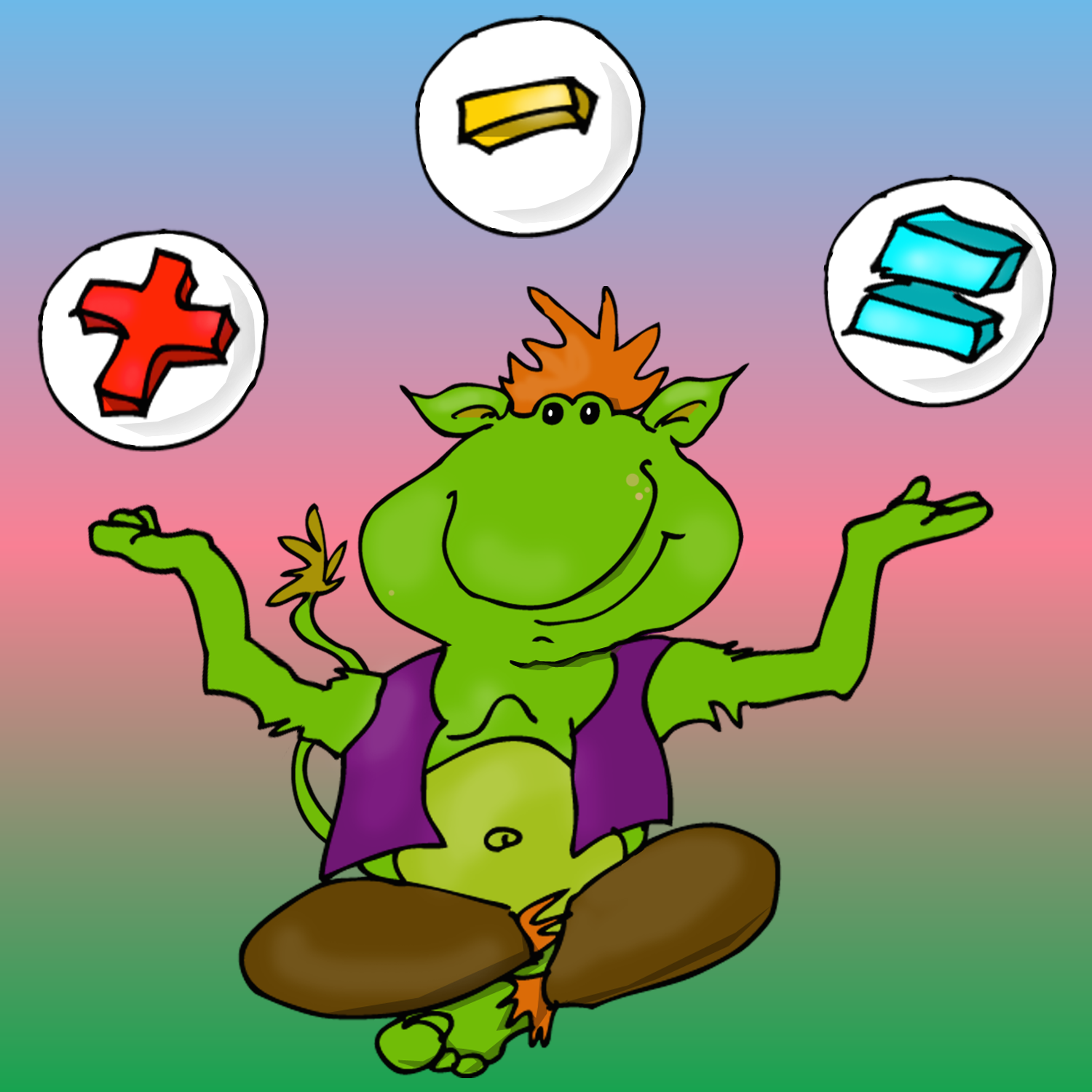en

# Practice MathsPractice Maths has been developed to provide a challenging and fun way to improve children's mathematical skills. The app is designed for children of early school age (preschool - grade 1) and is divided into 6 different levels, each containing 15 sublevels. The levels get harder while tasks will return to enhance the learning process. The following mathematical topics are included:

# Count the number (1-10).

# Count the number of legs of several figures (1-12).

# Friends of ten.

# Plus (from calculating figures to simpler terms).

# Minus (from calculating figures to simpler terms).

# Pattern (increases from simpler patterns with two different images to more difficult ones with 4 different pictures per pattern).

# Number sequences (increases from 1-20 all the way up to 100, 10s and even numbers).

# Work out your logical ability (which part of the image is missing and which shadow appears).

# Mathematical concepts (Fewest / most, lightest / heaviest, shortest / tallest, most / fewest / just as many).

# Geometric objects (circle, rectangle, square and triangle).

Practice Maths has been developed to be used by kids themselves to the highest possible extent. The setup (parents) menu is locked by a little more difficult mathematical problem. In this menu some configurations are possible along with the possibility to create and edit up to 10 different users, which is good if more than one kid uses the app. For each user, there is also the possibility to unlock all levels or to lock all levels to start from scratch again.